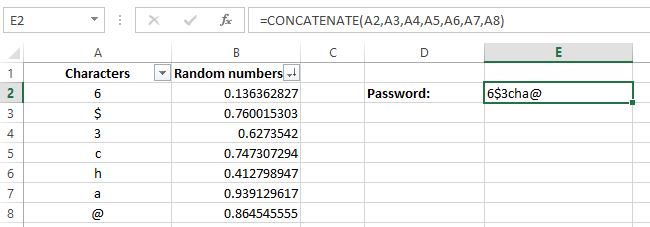# How To Randomize A List In ExcelSuppose you want to randomize a list, for this you have to have a list of random (or shuffled) numbers, which can be used to randomize the list you want. This may be useful in certain tasks to perform different activities, one of such example is described at the end of this tutorial.

This random number list can easily be created using one of the available excel functions (i.e. Random number formula).

The syntax used is explained below:

=Rand()

This is a very simple syntax, and it does not have any argument to be included. The function will simply return a random number between 0 and 1.

Create random number list

• Select one cell and type random number formula, =Rand()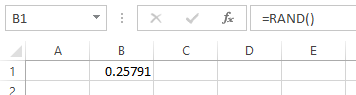• Then select the cell, click on the bottom right corner of the cell and drag down to create your list.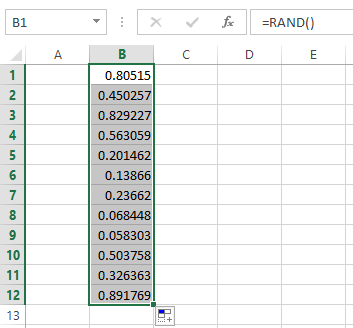• Now the random number list is created.

• But note that, these random numbers get refreshed every time a cell on the sheet is changed. To avoid this instant changes, you can coy the random number list and then paste them as values.

Randomize a list

Suppose you have a list of items and that you want it to be randomized. You can use this random number list to perform this task.

• Consider that you want to shuffle column A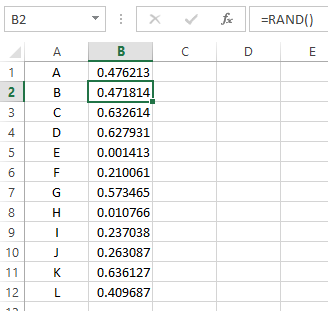• So create a random number list in column B, refresh it as you want

• Copy the random number list and past it as values, so no longer it get changed.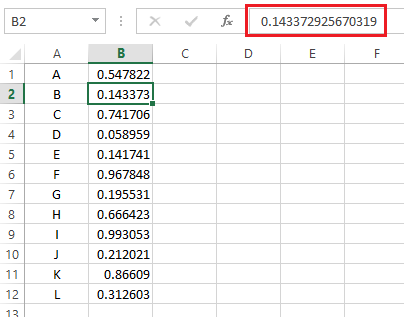• Go to Data tab > Sort the number list > you get a randomized list of data in column A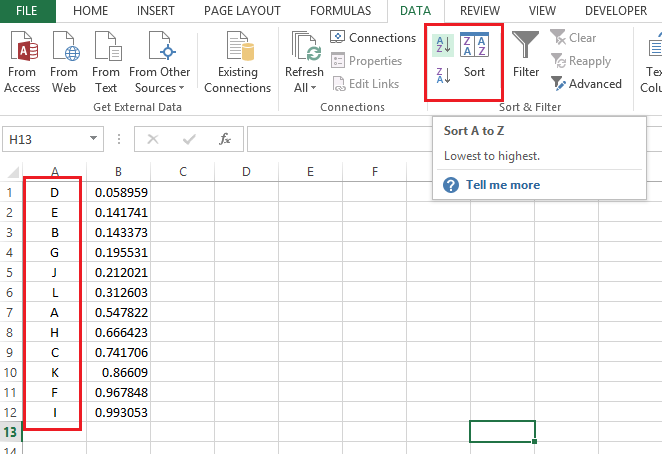Example #1

The random number list can be used to create a random password, using some characters. Random number list is used to shuffle the characters and then the password has been created combining the individual characters.